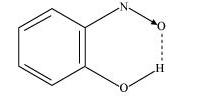# Define hydrogen bond. Is it weaker or stronger than the van der Waals forces?

Question:

Define hydrogen bond. Is it weaker or stronger than the van der Waals forces?

Solution:

A hydrogen bond is defined as an attractive force acting between the hydrogen attached to an electronegative atom of one molecule and an electronegative atom of a different molecule (may be of the same kind).

Due to a difference between electronegativities, the bond pair between hydrogen and the electronegative atom gets drifted far away from the hydrogen atom. As a result, a hydrogen atom becomes electropositive with respect to the other atom and acquires a positive charge.

$4^{\delta}-X^{\delta-} \ldots \ldots . H^{\delta+}-X^{\delta-} \ldots \ldots . . H^{\delta+}-X^{\delta-}$

The magnitude of H-bonding is maximum in the solid state and minimum in the gaseous state.

There are two types of H-bonds:

(i) Intermolecular H-bond e.g., HF, H2O etc.

(ii) Intramolecular H-bond e.g., o-nitrophenolHydrogen bonds are stronger than Van der Walls forces since hydrogen bonds are regarded as an extreme form of dipole-dipole interaction.